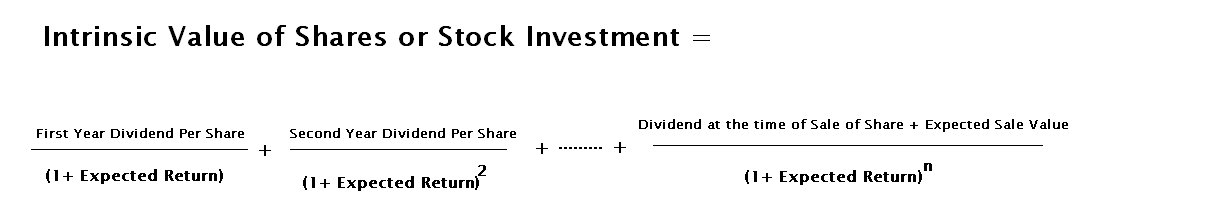222

# Why Accounting Education Important# Why Accounting Education Volunteer## Become Volunteer Teacher

Intrinsic value analysis is so important for every investor who wants to invest their money in share market and also want to increase his share investment wealth because this analysis will directorate the investor when will he or she buy the shares and when will he or she sell the shares?

It is the basic principle, when the market value of shares will be less than its intrinsic value, investor must buy the shares. When the market value is more than its intrinsic value, it is the proper time to sell the shares. I repeat again

(A) Buy Shares of A Company = When = Market Value <  Intrinsic Value

(B) Sell Shares of A Company = When =  Market Value > Intrinsic Value

In Intrinsic Value analysis, we will explain the meaning, steps to calculate the intrinsic value and other fundamental concepts of its analysis.

Meaning of Intrinsic Value

Intrinsic value of a company's stock is the real value which is calculated with fundamental analysis without calculating its market value. Forget what is the market value, just check the future earning's present value. When investor invests in the shares, it will be its fixed asset. Its value will be so high if it will generate high revenue within less period. Revenue of share investment is dividend. So, we will calculate the present value of future dividend for finding the intrinsic value of stock.

Steps to Calculate Intrinsic Value

Simple formula for calculating Intrinsic Value

We are explaining the calculating of intrinsic value with three examples

1. # Mr. A wants to return 30% on his stock investment for every year for 3 years. Company will give dividend (estimated) 30%, 40% and 50% on its face value \$ 10. So, he will receive the dividend first year \$ 3, second year \$ 4 and third year \$ 5. At the end of year, he can sold it on \$ 300

Intrinsic value will be

= 3/1.3 + 4 / (1.3)^2 + (5+300)/ (1.3)^3 = \$ 143.50

Intrinsic Value analysis in this case = If the market value of the share is below \$ 143.50, then the share is below its intrinsic value, therefore well worth purchasing. If, on the other hand, the market value is higher than intrinsic value \$ 143.50, it is the good time to sell.

2. # For different investors, intrinsic value may be different. For example,  Mr. A wants to return 30% on his stock investment for every year for 3 years. Company will give dividend (estimated) 30%, 40% and 50% on its face value \$ 10. So, he will receive the dividend first year \$ 3, second year \$ 4 and third year \$ 5. At the end of year, he can sold it on \$ 300 but Mr. B  wants to return 40% on his stock investment for every year for 3 years. Company will give dividend (estimated) 30%, 40% and 50% on its face value \$ 10. So, he will receive the dividend first year \$ 3, second year \$ 4 and third year \$ 5. At the end of year, he can sold it on \$ 300

Intrinsic Value for Mr. A = 3/1.3 + 4 / (1.3)^2 + (5+300)/ (1.3)^3 = \$ 143.50

Intrinsic Value for Mr. B =  3/1.4 + 4 / (1.4)^2 + (5+300)/ (1.4)^3

= \$ 115.33

Intrinsic Value analysis in this case = When the market value of share will reach \$ 143.50, Mr. A should keep it but Mr. B should sell for getting profit from his investment because Mr. B's intrinsic value is just \$ 115.33 which is less than its market value.

3. # The price of share of a company is \$ 170. Its profit in the year to 31st march 2013 had grown by 70% ( \$ 20 Million). Its earning per share (EPS) which was improvement of 25%. If we assume that in the next 2 years its EPS will grow by 30%. This company's share's market value at the end of 2nd year will be \$235. In 2013, company paid dividend of 25%. In 2014, it is the chance, company will pay the dividend of 30% and in 2015, it will give of 40%.

Intrinsic Value

= 3.0/1.3 +(4.0+235)/(1.3)^2 =  \$143.72

Intrinsic Value analysis in this case =  At 31st march 2013, market value of share is \$ 170 but today intrinsic value is \$ 143, so, investor who is expecting 30% return can sell the share because today market value is \$ 170 which is more than its intrinsic value.

But same investor is expecting just 10% return or company's EPS will grow just 10%, at that time intrinsic value will be

= 3.0/1.1 +(4.0+235)/(1.1)^2 =  \$200.24

So, in this case, investor must not sell his shares because his intrinsic value is more than current
market value.

References :

1. Finance Book (Topic Fundamental Analysis)
2. http://en.wikipedia.org/wiki/Intrinsic_value_(finance): 1
1.hello,sir, today i read more deeply intrinsic value and understand it.thanks

In comment, you can give your feedback, reviews, ideas for improving content or ask question relating to written content.

Name

ltr
item
Accounting Education: Intrinsic Value Analysis
Intrinsic Value Analysis
http://4.bp.blogspot.com/-FCM6DwV3n0w/UubYIp7PvUI/AAAAAAAAMsk/-Hjmdi2Dan0/s1600/intrinsic+value.PNG
http://4.bp.blogspot.com/-FCM6DwV3n0w/UubYIp7PvUI/AAAAAAAAMsk/-Hjmdi2Dan0/s72-c/intrinsic+value.PNG
Accounting Education
https://www.svtuition.org/2014/01/intrinsic-value-analysis.html
https://www.svtuition.org/
http://www.svtuition.org/
http://www.svtuition.org/2014/01/intrinsic-value-analysis.html
true
2410664366776677676
UTF-8